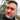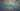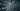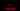razorborg

# Long ints from data streams in JavascriptJan Martin Borgersen
September 2nd, 2015 · 3 min readThis was interesting, and I wasnt able to find this specific issue discussed online, so here I go attempting to add to the general knowledge of the Internet.

The challenge: In Javascript, read 8 bytes out of an array (for instance, a file or a network packet) and turn it into a number. 53-bit precision is okay (Javascript stores numbers as 64-bit floats with a 53-bit mantissa). Use Uint8Array as our view into the byte array.

Other languages have 64-bit long ints, and this is straightforward. Other languages also have uints, which simplify dealing with 1s in the most significant bit. However, Javascript treats all numbers as floats with 53-bit precision (the remaining 11 bits are exponent). To get around this, there are a variety of Big Integer libraries that have popped up to enable 64-bit or string-backed arbitrary length large numbers. However, Im working with code that began its life in Java, and still has to work in Java, so I dont want to refactor much. Also, 53-bit precision is okay for this problem because these numbers are really only timestamps. I want to handle this math in an abstraction layer that gives me a regular Javascript number to use elsewhere.

## The classic approach: bit shifting

The bit shifting approach works great in languages with 64-bit long ints and looks something like this:

`.css-5nn1ka{position:absolute;right:22px;top:24px;padding:8px 12px 7px;border-radius:5px;color:#6f7177;-webkit-transition:background 0.3s ease;transition:background 0.3s ease;}.css-5nn1ka:hover{background:rgba(255,255,255,0.07);}.css-5nn1ka[data-a11y="true"]:focus::after{content:"";position:absolute;left:-2%;top:-2%;width:104%;height:104%;border:2px solid var(--theme-ui-colors-accent,#3eb0ef);border-radius:5px;background:rgba(255,255,255,0.01);}@media (max-width:45.9375em){.css-5nn1ka{display:none;}}1for (var i = 0; i < 8; i++) {2    if (i > 0) num <<=8;3    num |= arr[i] & 0xFF;4}`

However, bit shifting in Javascript turns numbers into 32-bit integers (they fit nicely inside a 64 bit float). So this approach shifts your most significant four bytes out of the number entirely.

This wont work for us.

## The higher-level math approach: multiply and add

Multiplying the number by 256 (0x100) and adding the next byte is the mathematical equivalent of left-shifting by 8, and we wont lose the upper 21 bits of precision. Moreover, since bit-shifting doesnt buy us any better performance in Javascript, using regular math is just fine. This approach looks like this:

`1for (var i = 0; i < 8; i++) {2    if (i > 0) num *= 0x100;3    num += arr[i] & 0xFF;4}`

However, this produces some very wrong results when the number is negative and padded with 1s all the way to the left. After enough runs through the loop, `num` starts to look negative, and adding `arr[i]` to a negative number moves `num` in the wrong direction.

## The two 32-bit ints approach

I eventually decided that it was a safer to read the 8 bytes into two 4 byte number words and calculate the correct 53-bit number. This way I wasnt going to lose any bits until I needed to throw away the upper 21 bits.

Much like the multiply-by-256-and-add approach, this works great (and easily) for positive numbers:

`1for (var i = 0; i < 8; i++) {2    if (i < 4) {3        if (i > 0) high = high * 0x100;4        high += arr[i] & 0xFF;5    } else {6        if (i > 4) low = low * 0x100;7        low += arr[i] & 0xFF;8    }9}10num = high * 0x100000000 + low;`

However, negatives are a problem now because were only using the bottom 32 bits of a 53-bit float mantissa, so neither the high nor the low int word look like negative numbers until that multiplication step. (Remember this, itll be important again later.) During the multiplication, if `high * 0x100000000` gives us a 1 in bit 53, it suddenly looks negative to Javascript, adding a positive `low` value to it, well, its the wrong answer.

We have to be smarter.

## Two’s Complement Math

Negative numbers are represented in twos complement form, which, if you remember your CS101, gives us one extra number of representation than simply using a sign bit. It also makes for some elegant bit-level math. But were in Javascript, so bit-level math isnt elegant anymore; in fact, it keeps getting us into trouble here.

Converting to a twos complement number is simple on paper: flip the bits and add one. Now you have the negative representation of your value. So this feels like it should work:

`1if (high >= 0x80000000) { // see if 64-bit number is negative2   high ^= 0xFFFFFFFF;3   low ^= 0xFFFFFFFF;4   num = -(high * 0x100000000 + low + 1);5}`

But heres where Javascript gets weird again, and its all about bit-level math suddenly turning numbers into 32-bit signed ints. Fire up a console or node and try this:

`1> var num = 123;2> num ^= 0xFFFFFFFF;3-124`

See that negative number? If you flip the bits on the low word and theres a 1 at bit 32, the number goes negative. `high * 0x100000000 + low` is now a subtraction instead of an addition, and we get the wrong answer. We have the same problem we hit the last time we tried this line of code, except this time `low` is the negative value.

## Special case the low word

So the way around this is to make sure, when we flip the bits of the low word, we never have a negative number. This seems to work:

`1if (high >= 0x80000000) {2    high ^= 0xFFFFFFFF;3    var lowIsPositive = false;4    if (low < 0)5        low ^= 0xFFFFFFFF;6    else {7        lowIsPositive = true;8        low ^= 0x7FFFFFFF;9    }10    num = -(high * 0x10000000011        + low12        + 113        + (lowIsPositive ? 0x80000000 : 0));14else15    num = high * 0x100000000 + low;`

One curious note: the last line of code still works without worrying about the sign of `low` because, when we read the bytes into the number, we used the multiply-and-add approach instead of the bit-shift approach. Even if the 32nd bit of `low` is a 1, it is still considered a positive number. Until, of course, we do some bitwise math and Javascript turns it into a 32 bit signed int.

## Morals

My takeaways from this exercise are:

• Sometimes understanding number representation is even more important in high-level languages like Javascript than in low-level languages like C
• Mixing bitwise and numeric math in Javascript is dangerous; be careful

### More articles from Jan Martin Borgersen### Fun takeaways from the 2014 Internet Trends Report

… on user focus: http://techcrunch.com/gallery/mary-meeker-internet-trends/slide/14 Users want quick, straight-forward apps that nail a…

May 31st, 2014 · 2 min read### Fun talk from a couple of weeks ago at Netflix about Reactive Extensions in Javascript.

March 29th, 2014 · min read